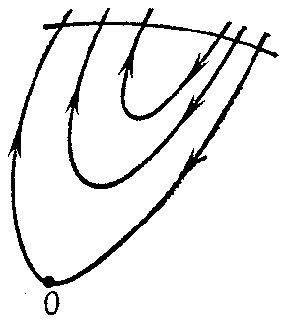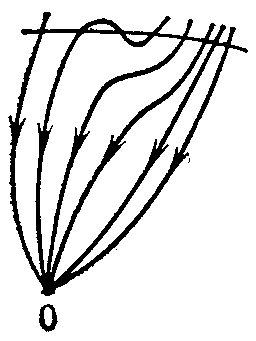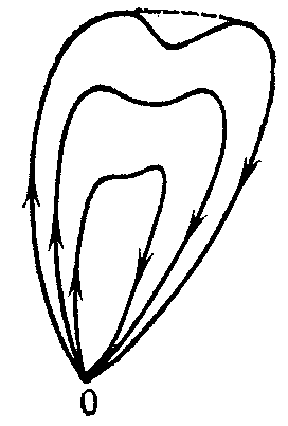# Sector in the theory of ordinary differential equations

An open curvilinear sector $S$ with vertex at an isolated singular point $O$ of an autonomous system of second-order ordinary differential equations

$$\tag{* } \dot{x} = f ( x),\ \ x \in \mathbf R ^ {2} ,$$

$f \in C ( G)$, where $G$ is the domain of uniqueness, that satisfies the following four conditions: 1) each lateral boundary of $S$ is a $TO$- curve of the system (*) (i.e. a semi-trajectory that approaches $O$ as $| t | \rightarrow + \infty$, and touches a certain direction at $O$); 2) the outer boundary of $S$ is a simple parametric arc (the homeomorphic image of a closed interval); 3) $\overline{S}\; \setminus \{ 0 \}$ does not contain singular points of (*). The fourth condition is one of the following three: 4a) all trajectories of the system (*) that start in $S$ leave this sector for both increasing and decreasing $t$; such a sector is called a hyperbolic sector, or a saddle sector (Fig. a); 4b) all trajectories of (*) that start in $S$ sufficiently near $O$ do not leave $S$ but approach $O$ as $t$ increases, and as $t$ decreases they leave $S$( or vice-versa); such a sector is called a parabolic sector or an open node sector (Fig. b); or 4c) all the trajectories of (*) that start in $S$ sufficiently near $O$ do not leave $S$ as $t$ increases or decreases but approach $O$, forming together with $O$ closed curves (loops), and for any two loops one encloses the other; such a sector is called an elliptic sector or a closed node sector (Fig. c).Figure: s083770aFigure: s083770bFigure: s083770c

For any analytic system (*) with $TO$- curves, a disc $Q$ of sufficiently small radius and centre at $O$ can always be divided into a finite number of sectors of a specific form: $h$ hyperbolic, $p$ parabolic and $e$ elliptic ones (see  and ). The Frommer method can be used to exhibit all these sectors, to determine the type of each, and to establish the rules of their succession in a circuit about $O$ along the boundary of $Q$( and thereby to show the topological structure of the arrangement of the trajectories of (*) in a neighbourhood of $O$). There are a priori estimates from above for $h$, $p$ and $e$ in terms of the order of smallness of the norm $\| f ( x) \|$ as $x \rightarrow 0$( see , , ).

Sometimes (see, for example, ) the notion of a "sector" is defined more freely: In hyperbolic and parabolic sectors loops are allowed that cover a set without limit points on the rear boundary of a sector, and in elliptic sectors, loops that do not contain one another. Here the first sentence of the previous paragraph remains valid also for a system (*) of general form, and the Poincaré index $i$ of the singular point $O$ of (*) is expressed by Bendixson's formula

$$i = 1 + \frac{e - h }{2} .$$

How to Cite This Entry:
Sector in the theory of ordinary differential equations. Encyclopedia of Mathematics. URL: http://encyclopediaofmath.org/index.php?title=Sector_in_the_theory_of_ordinary_differential_equations&oldid=48642
This article was adapted from an original article by A.F. Andreev (originator), which appeared in Encyclopedia of Mathematics - ISBN 1402006098. See original article